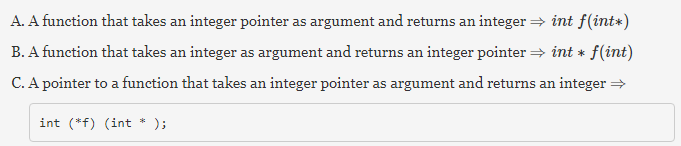# Gate CS-2005 Question Paper With Solutions

Q. 1 What does the following C-statement declare?

``int` `( * f) (``int` `* ) ;``

(A) A function that takes an integer pointer as argument and returns an integer.

(B) A function that takes an integer as argument and returns an integer pointer.

(C) A pointer to a function that takes an integer pointer as argument and returns an integer.

(D) A function that takes an integer pointer as argument and returns a function pointer